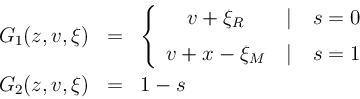### Theory-based implementation

• Implementation of version C as probabilistic state space model:
• functions are time-dependent (full/half year)
• standard trick: z = (x, s)'
• s = 0/1 at full/half year → s(k+1) = 1 - s(k)
• define G, H and ξ, e.g.
•• Simulink implementation tedious, but completely routine
• replace TimeSwitch by PhaseSwitch using s
• example G(z, v, ξ)
•• complete school model
• uses Sum instead of TimeSwitch
• has sample time 1/2 everywhere